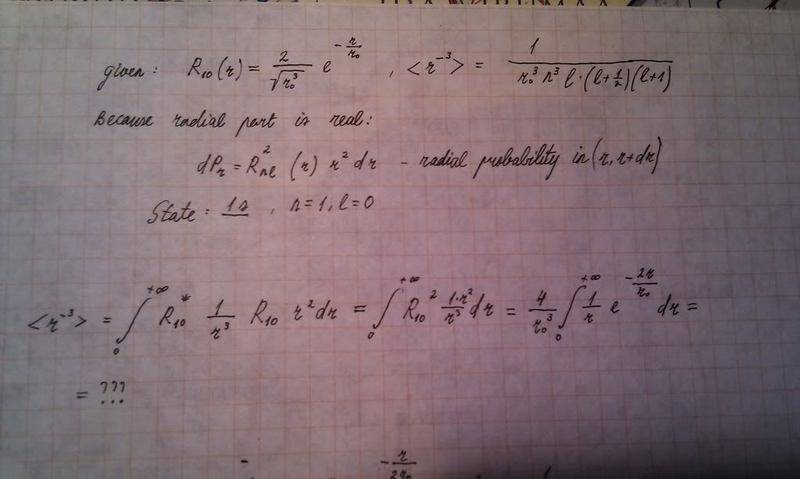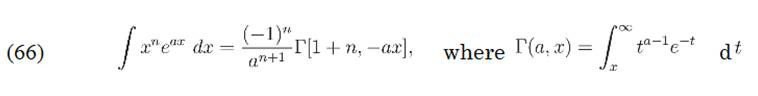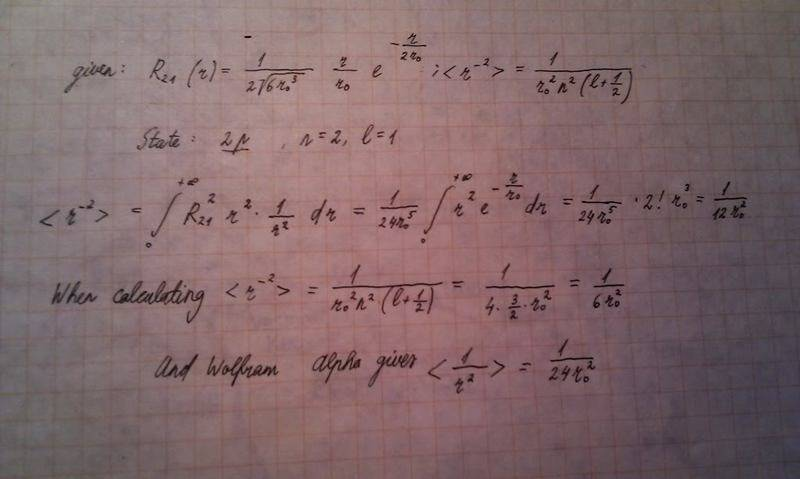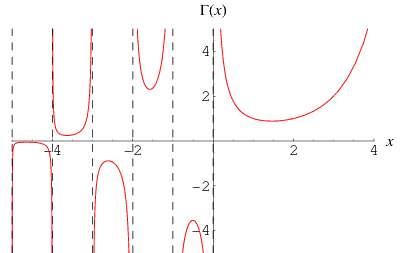# Why 1/(r^3) exp. value for H-atom diverges for 1s state? please respond before Jan 6

Hydrogen atom: 1/(r^2 )2p state constant problem //1/(r^3) exp. value

## Homework Statement

Hello, I am new to this forum. I hope it is all right I uploaded problems as image files (in handwriting).

I calculated all (r^(-1), r^(-2) and r^(-3) expectation values for Hydrogen atom for 1s and 2p. Almost all calculations gave the required result - the same as the theoretical formulas with quantum numbers, but r^(-3) for 1s and 2s state give an infinity when calculating using quantum numbers and should give the same when integrating. My question: Why does this integral diverge?
The first problem:I found out that there is a similar formula on integral-table.combut I guess there n is a natural number, am I right?

## Homework Statement

r^(-2) for 2p - there I have a constant problem:## The Attempt at a Solution

Last edited:

from the simple fact that $$\Gamma(z+1) = z\Gamma(z)$$ where $$\Gamma(z) = \int_{0}^{+ \infty} t^{z-1} e^{-t} dt$$

Yeah, I knew it would settle down for Gamma function. If I got it then:

When to reparametrize r=r0t and calculate, we get the definition of Gamma function. Because in my problem, n was -1, I would have to calculate Gamma(-1). It's modulus is +infinity, because Gamma(n)= [Gamma(n+2)]/[n*(n+1)] and if n=-1, then we get division by zero and it's limit is infinity.

I hope that is more or less it? Can anybody validate this?

The constant problem still stands.

I think understand the divergence problem, quite simple actually. Integrating by parts gives Gamma(0), because one can show, that u*v limits to 0, so no recurrence relation for Gamma function needed at all, because modulus of Gamma(0) tends to infinityPS! I was also right at the beginning. It can be also done by the upper incomplete Gamma function. Consider the formula:What matters is on the right - I get the special case for upper incomplete gamma function (0,0) that in fact equals to Gamma(0) and this limit tends to infinity. At the same time this is also the special case for the lower incomplete gamma function, so they coincide for 0.
As you can read from http://mathworld.wolfram.com/IncompleteGammaFunction.html
and http://en.wikipedia.org/wiki/Incomplete_gamma_function the incomplete gamma function is the generalized gamma function.

The second problem is more fundamental now - actually I had differences (*1/2) in 2 cases. In one case, Alpha got the same answer as me (integrating), but theoretical formula differs by *1/2.
The other one is the one of which I have the second picture here - there I had a mistype, now Alpha gives the same answer there too, but the result with theoretical formula still differs *1/2.

So now the problem is - the theoretical formula with quantum numbers doesn't give the same answer as my integral and Wolfram Alpha!

Can anybody give me an insight what is going on? I don't know if I should find any similar formulas from a textbook, these formulas I took from professor's notes.

Thanks!

Last edited:

I figured even integration by parts wasn't necessary, only a change of variable.

I passed the exam and the professor did not find any mistakes in my integrals.

There is a chance that at least r^(-2) expectation value formula with quantum numbers may be slightly faulty. I did not have time to check them before the exam but the professor promised to check and maybe some day I will know where the rub was.